Courses
Courses for Kids
Free study material
Offline Centres
More

# NCERT Exemplar for Class 11 Chemistry Chapter-5 (Book Solutions)Last updated date: 07th Dec 2023
Total views: 612.3k
Views today: 17.12k

## NCERT Exemplar for Class 11 Chemistry - States of Matter - Free PDF Download

Free PDF download of NCERT Exemplar for Class 11 Chemistry Chapter 5 - States of Matter solved by expert Chemistry teachers on Vedantu.com as per NCERT (CBSE) Book guidelines. All Chapter 5 - the States of Matter exercise questions with solutions to help you to revise the complete syllabus and score more marks in your Examinations.

Download the free PDF of NCERT Exemplar that is designed for Class 11 students from the website of Vedantu. A detailed overview of the Chapters along with solved question papers and sample question papers are included in these books. To make your learning process even better, Vedantu has taken up this initiative to provide the students with free course materials that can be downloaded, so that even when the internet connection is down, it does not create any hindrance in the way of the students getting prepared for the Examinations.

## Access NCERT Exemplar Solutions for Class 11 Chemistry Chapter – 5 States of Matter

### I. Multiple Type Questions (Type I):

1. A person living in Shimla observed that cooking food without using pressure cooker takes more time. The reason for this observation is that at high altitude:

i. pressure increases

ii. temperature decreases

iii. pressure decreases

iv. temperature increases

Ans: iii. pressure decreases

At high altitude the pressure is low and therefore, water boils at lower temperature because the vapour pressure becomes equal to atmospheric temperature at low temperature due to which the food takes more time to be cooked without using a pressure cooker.

2.  Which of the following property of water can be used to explain the spherical shape of rain droplets?

i. viscosity

ii. surface tension

iii. A critical phenomena

iv. pressure

Ans: ii surface tension

Surface tension minimises the surface area of the liquid and at  minimum surface area, the liquid is in its lowest energy state and hence most stable. Spherical shape of rain droplets has minimum surface area due to surface tension.

3. A plot of volume (V) versus temperature (T) for a gas at constant pressure is a straight line passing through the origin. The plots at different values of pressure are shown in Fig. 5.1. Which of the following order of pressure is correct for this gas?i. p1 > p2 > p3 > p4

ii. p1 = p2 = p3 = p4

iii. p< p2 < p3 < p4

iv. p< p2 = p3 < p4

Ans: iii. p< p2 < p3 < p4

At a particular temperature, PV is constant

Therefore,  V ∝  $\dfrac{1}{p}$

So, as v1 >  v2  >  v3  >  v4     the order of pressure:    p< p2 < p3 < p4 .

4. The interaction energy of London force is inversely proportional to sixth power of the distance between two interacting particles but their magnitude depends upon

i. charge of interacting particles

ii. mass of interacting particles

iii. polarisability of interacting particles

iv. strength of permanent dipoles in the particles.

Ans: iii. polarisability of interacting particles.

The energy of the London force or London dispersion force  is inversely proportional to (distance between two interacting particles)6 but their magnitude depends upon the polarisability of interacting particles.

5. Dipole-dipole forces act between the molecules possessing permanent dipole. Ends of dipoles possess 'partial charges'. The partial charge is

i. more than unit electronic charge

ii. equal to unit electronic charge

iii. less than unit electronic charge

iv. double the unit electronic charge

Ans: iii. less than unit electronic charge

Charge of 1 electron is 1.6 × 10 -19 C and the partial charge is always less than the unit electronic charge.

6. The pressure of a 1:4 mixture of dihydrogen and dioxygen enclosed in a vessel is one atmosphere. What would be the partial pressure of dioxygen?

i. 0.8 × 10 5 atm

ii. 0.008 Nm-2

iii. 8 ×104  Nm-2

iv. 0.25 atm

Ans: iii. 8 × 104  Nm-2

Partial pressure of oxygen, $\text{P}{{\text{o}}_{2}}=\chi {{\text{o}}_{\text{2}}}\times {{\text{P}}_{\text{total}}}$

Mole fraction of O2 ($\chi$O2 ) =     $\dfrac{\text{Moles }\!\!~\!\!\text{ of }\!\!~\!\!\text{ Oxygen}}{\text{Moles }\!\!~\!\!\text{ of }\!\!~\!\!\text{ oxygen }\!\!~\!\!\text{ + }\!\!~\!\!\text{ Moles }\!\!~\!\!\text{ of }\!\!~\!\!\text{ hydrogen}}$

$~~~$       =   $\dfrac{4}{4+1}~~=~$ $\dfrac{4}{5}$

PO2  =  $\dfrac{4}{5}$×1 = $\dfrac{4}{5}$ 0.8 atm              (∵Ptotal = 1 atm)

1 atm =  1.1034 × 105 Nm-2 or Pa

$\therefore$  Partial pressure of dioxygen = 0.8 ×105 = 8×10Nm-2

7. As the temperature increases, average kinetic energy of molecules increases. What would be the effect of increase of temperature on pressure provided the volume is constant?

i. increases

ii. decreases

iii. remain same

iv. becomes half

Ans:  i.  increases

Gay Lussac’s law shows the direct relationship between the pressure and the temperature of a fixed amount of gas at constant volume.

Gay Lussac's law states that at constant volume, the pressure of a fixed amount of a gas is directly proportional to the temperature.

Therefore,

P∝T                          ………..at constant volume

Thus, pressure increases if the temperature is increased at a constant volume.

To elaborate more on this topic, we will see a real-life example.

8. Gases possess characteristic critical temperature which depends upon the magnitude of intermolecular forces between the particles. Following are the critical temperatures of some gases.

 Gases Critical temperature in Kelvin H2 33.2 He 5.3 O2 154 .3 N2 126

From the above data what would be the order of liquefaction of these gases? Start writing the order from the gas liquefying first

i. H2, He, O2, N2

ii. He, O2,  H2, N2

iii. N2, O2, He, H2

iv. O2, N2, H2, He

Ans: iv. O2, N2, H2, He

Higher the critical temperature of gases more easily, the gas will be liquefied. So, the order of liquefying of gases will be  O2 > N2 > H2 >He.

9. What is SI unit of viscosity coefficient?

i. Pascal

ii. Nsm-2

iii. km-2

iv. Nm-2

Ans: ii. Nsm-2

Viscosity coefficient ($\text{ }\!\!\eta\!\!\text{ }$) =  $\dfrac{\text{Force}}{\text{Area }\!\!~\!\!\text{ }\!\!~\!\!\text{ }\times \text{ }\!\!~\!\!\text{ }\!\!~\!\!\text{ velocity }\!\!~\!\!\text{ gradient}}$

SI unit of $\text{ }\!\!\eta\!\!\text{ }$ = Nsm-2

10. Atmospheric pressures recorded in different cities are as follows:

 Cities p in N/m-2 Shimla 1.01 ×105 Bangalore 1.2 × 105 Delhi 1.02 × 105 Mumbai 1.21 ×105

Consider the above data and mark the place at which liquid will boil first.

i. Shimla

ii. Bangalore

iii. Delhi

iv. Mumbai

Ans: i. Shimla

The temperature at which the liquid boils when the vapour pressure becomes equal to the atmospheric pressure  is known as the boiling point of the liquid. When the  atmospheric pressure is low, the boiling point of the liquid will be low. As Shimla has the lowest atmospheric pressure, it will boil first.

11. Which curve in Fig. 5.2 represents the curve of ideal gas?

i. B only

ii. C and D only

iii. E and F only

iv. A and B onlyAns:  i. B only

For all ideal gas PV = constant.  Only B has no change with the change in PV, so only line B represents the curve of ideal gas.

12. Increase in kinetic energy can overcome intermolecular forces of attraction. How will the viscosity of liquid be affected by the increase in temperature?

i. Increase

ii. No effect

iii. Decrease

iv. No regular pattern will be followed

Ans: iii. Decrease

The increase in temperature increases the kinetic energy which can overcome intermolecular forces of attraction due to which the viscosity decreases and the liquid starts flowing.

13. How does the surface tension of a liquid vary with increase in temperature?

i. Remains same

ii. Decreases

iii. Increases

iv. No regular pattern is followed

Ans: ii.  Decreases

When the temperature increases, kinetic energy of the molecule increases which leads to decrease in the intermolecular forces and hence, decreases the surface tension.

### II. Multiple Choice Questions (Type-II)

In the following questions two or more options may be correct.

14. With regard to the gaseous state of matter which of the following statements are correct?

i. Complete order of molecules

ii. Complete disorder of molecules

iii. Random motion of molecules

iv. Fixed position of molecules

Ans: ii. Complete disorder of molecules and iii. Random motion of molecules

In a gaseous state of matter, molecules are arranged in complete disorder and  they move in random motion in all directions.

15. Which of the following figures does not represent 1 mole of dioxygen gas at STP?

i. 16 grams of gas

ii. 22.7 litres of gas

iii. 6.022 × 1023 dioxygen molecules

iv. 11.2 litres of gas

Ans: i.  16 grams of gas and iv. 11.2 litres of gas

1 mole of dioxygen at STP  occupies   =  22.4 L

=  molar mass of dioxygen    =   32 g

=  contain  6.023×1023 molecules of  oxygen gas

16. Under which of the following two conditions applied together  a gas deviates most from the ideal behaviour?

i. Low pressure

ii. High pressure

iii. Low temperature

iv. High temperature

Ans: ii. High pressure and iii. Low temperature

The real gases obey the ideal gas equation, PV= nRT at low pressure and high temperature. So, a gas does not obey the ideal gas equation or behave ideally at high pressure and low temperature.

17. Which of the following changes decrease the vapour pressure of water kept in a sealed vessel?

i. Decreasing the quantity of water

iii. Decreasing the volume of the vessel to one-half

iv. Decreasing the temperature of water

Ans: ii. Adding salt to water and iv. Decreasing the temperature of water

By adding salt to water,  the water molecule available at the surface to evaporate will be low and hence, the vapour pressure decreases. Also vapour pressure is directly proportional to temperature so when we decrease the temperature of water the vapour pressure decreases.

18. If 1 gram of each of the following gases are taken at STP. which of the gases will occupy

(a) greatest volume and (b) smallest volume?

CO,           H2O,            CH4,                NO

Ans:  (a) greatest volume is occupied by CH4 and (b) smallest volume by NO

Volume of 1 mole of a gas at STP   =   22.4 L

Now , we know,

1 mole    =     Molar mass

∴   Volume of 28g mol-1 of CO at STP   =   22.4 L

So, Volume of 1 g  of CO at STP   =   22.4 L / 28 g mol-1

Similarly,

Volume of 1 g  of  H2O at STP   =   22.4 L / 18 g mol-1

Volume of 1 g  of  CH4 at STP   =   22.4 L / 16 g mol-1              (Greatest volume)

Volume of 1 g  of  NO at STP    =   22.4 L / 30 g mol-1               (smallest volume)

19. Physical properties of ice, water and steam are very different. What is the chemical composition of water in all the three states?

Ans: Physical state of ice, water and steam are very different but the chemical composition of water in all the three states is H2O.

20. The behaviour of matter in different states is governed by various physical laws. According to you what are the factors that determine the state of matter?

Ans: The factors that determine the states of matter are:

➔ Temperature

➔ Pressure

➔ Mass and volume

21. Use the information and data given below to answer the questions (a) to (c):

Stronger intermolecular forces result in higher boiling point.

Strength of London forces increases with the number of electrons in the molecule.

Boiling point of HF, HCI, HBr and HI are 293 K, 189 K, 206 K and 238 K respectively.

a. Which type of intermolecular forces are present in the molecules HF, HCI, HBr and HI?

Ans: HCl, HBr and HI have dipole-dipole and london dispersion  interaction   whereas HF has hydrogen bonding in addition (due to the high electronegativity of the F atom).

b. Looking at the trend of boiling points of HCl, HBr and HI, explain out of dipole-dipole interaction and London interaction, which one is predominant here.

Ans: The electronegativity decreases from Cl, Br and I so the dipole-dipole interaction will also decrease as HCl > HBr > HI  and it is contrary to the boiling point order which is HCl < HBr < HI. This confirms that the London interaction is predominant.

c. Why is the boiling point of hydrogen fluoride highest while that of hydrogen chloride lowest?

Ans: F atom has highest electronegativity so it has hydrogen bonding interaction in addition to   dipole-dipole and london dispersion  interaction which leads to the highest boiling point of HF whereas  HCl has lowest boiling point  because london force plays  the predominant role in boiling point than dipole-dipole interaction and  london force increases proportionally with the molecular weight of the compound. HCl has lowest molecular weight among HCl, HBr and HI therefore it has the least london force interaction which leads to the lowest boiling point.

22. What will be the molar volume of nitrogen and argon at 273.15 K and 1 atm?

Ans: The molar volume of both nitrogen and argon at 273.15 K and 1 atm is 22.4 L.

At STP (1 atm pressure and 273.15 K or 00 C), the molar volume i.e the volume of 1 mole gas is 22.4L.

23. A gas that follows Boyle's law, Charle's law and Avogadro's law is called an ideal gas. Under what conditions a real gas would behave ideally?

Ans: A gas that  follows Boyle's law, Charle's law and Avogadro's law is called an ideal gas.

Real gas behaves ideal under low pressure and high temperature. Under these conditions, the intermolecular interactions are minimum.

24. Two different gases 'A' and 'B' are filled in separate containers of equal capacity under the same conditions of temperature and pressure. On increasing the pressure slightly the gas 'A' liquefies but gas B does not liquify even on applying high pressure until it is cooled. Explain this phenomenon.

Ans:   On increasing the pressure slightly the gas 'A' liquefies but gas B does not liquify even on applying high pressure until it is cooled at same  conditions of temperature and pressure  filled in equal capacity containers it happens because   the gas ‘A’ being at the  critical temperature liquifies on slightly increasing the pressure and  gas ’B’ being at higher temperature than critical temperature does not liquifies even on applying high pressure until it is cooled.

25. Value of universal gas constant (R) is same for all gases. What is its physical significance?

Ans: Unit of R depends on the  units of  p, V and T are measured, We know,   R=$\dfrac{\text{pV}}{\text{nT}}$

Now, let's say, the  pressure is measured in Pascal, per mole volume is measured in m3 and temperature is measured in Kelvin,  then. Units of ‘R’ are Pa m3K-1 mol-1. Also, R is work done per mole per kelvin. It’s unit is J K-1 mol-1 (Joule is the unit of work done).

26. One of the assumptions of kinetic theory of gases states that "there is no force of attraction between the molecules of a gas." How far is this statement correct? Is it possible to liquefy an ideal gas? Explain.

Ans: The assumption   of the kinetic theory of gases which states that- “ there is no force of attraction between the molecules of a gas. ” is a correct statement. Ideal gas has minimum force of attraction between the molecules and hence, it cannot be liquified.  Liquefaction of a gas can only  be done when the force of attraction between the molecules increases, which is not possible for an ideal gas.

27. The magnitude of surface tension of liquid depends on the attractive forces between the molecules. Arrange the following in increasing order of surface tension :

water,  alcohol (C2H5OH) and hexane (CH3(CH2)4CH3)).

Ans:  Water and alcohol are polar  molecules which have high electronegative O atm  so they  have hydrogen bonding along with dipole-dipole interaction.   Hexane is a nonpolar molecule that has London dispersion force  which is a weak force between the molecules. Water has stronger H-bonding than alcohol. So, the order of attractive force will be:

Hexane  <   Alcohol  <  Water

More attractive forces between the molecules, the greater the  magnitude of surface tension of the liquid.

28. Pressure exerted by saturated water vapour is called aqueous tension. What correction term will you apply to the total pressure to obtain pressure of dry gas?

Ans: The gas collected over water, is moist and has saturated water vapour that exerts pressure which is known as aqueous tension. So the total pressure of the gas will be:

Pmoist gas   =   P dry gas      +    Aqueous Tension

∴    PDry  gas    =   P moist  gas    -    Aqueous Tension     or

PDry  gas    =   P Total         -    Aqueous Tension

So, the correction term you apply to the total pressure to obtain pressure of dry gas is  P Total  - Aqueous Tension.

29. Name the energy which arises due to motion of atoms or molecules in a body. How is this energy affected when the temperature is increased?

Ans:  The energy which arises due to motion of atoms or molecules in a body is known as thermal energy and the average kinetic energies of all the molecules increases with increases in temperature.

30. Name two intermolecular forces that exist between HF molecules in liquid state.

Ans:  The two intermolecular forces that exist between HF molecules in liquid state are hydrogen bonding (as F is a highly electronegative atom) and  dipole-dipole interaction (as HF is a polar molecule).

31. One of the assumptions of kinetic theory of gases is that there is no force of attraction between the molecules of a gas.

State and explain the evidence that shows that the assumption is not applicable for real gases.

Ans: The assumption of kinetic theory of gases that there is no force of attraction between the molecules of a gas is applicable for real gases. The evidence for this is  that the real gas can be liquified at high pressure and low temperature. This proves that there is force of attraction between the molecules of gas.

32. Compressibility factor, 2, of a gas is given as Z = $\dfrac{\text{PV}}{\text{nRT}}$

I. What is the value of Z for an ideal gas?

Ans: The value of Z for an ideal gas is 1.

II. For real gas what will be the effect on value of Z above Boyle's temperature?

Ans: For real gas, the value of Z > 1 above Boyle's temperature

33. The critical temperature (Tc) and critical pressure ( pc) of CO2 are 30.98°C and 73 atm respectively. Can CO2 (g) be liquefied at 32°C and 80 atm pressure?

Ans:  The CO2 gas cannot be liquified at the 32°C and 80 atm pressure as the given temperature and pressure are above critical temperature (Tc) and critical pressure (pc) which are 30.98°C and 73 atm respectively.

34. For real gases the relation between p, V and T is given by van der Waals equation:

P   +   $\dfrac{\text{a}{{\text{n}}^{\text{2}}}}{{{\text{V}}^{\text{2}}}}$ (V-nb) = nRT

where 'a' and are van der Waals constants, 'nb' is approximately equal to the total volume of the molecules of a gas.

'a' is the measure of magnitude of intermolecular attraction.

I. Arrange the following gases in the increasing order of 'b'. Give reason. O2, CO2,  H2, He

Ans:  The increasing order of  ‘b’ of the given gases is:

H2 <  He <  O2  < CO2

Volume of a gas is proportional to the size of the molecule.

II. Arrange the following gases in the decreasing order of magnitude of ‘a’. Give reason. CH4, O2, H2

Ans:  The decreasing order of magnitude of ‘a’ of the given molecule is:

CH4  >  O2  >  H2

The Van der waals constant ‘a’ represents the magnitude of intermolecular attraction which increases with increase in the size of the electron cloud  of the molecule. So the greater the size of a molecule, greater will be the electron cloud and hence greater will be the polarisability and more will be the magnitude of intermolecular attraction.

35. The relation between pressure exerted by an ideal gas (pideal) and observed pressure (preal) is given by the equation:

Pideal  =  Preal   + $\dfrac{a{{n}^{2}}}{{{V}^{2}}}$

If pressure is taken in Nm-2,  number of moles in mol and volume in m3, Calculate the unit of 'a'.

What will be the unit of 'a' when pressure is in atmosphere and volume in  dm3?

Ans: Pideal  =  Preal   + $\dfrac{a{{n}^{2}}}{{{V}^{2}}}$

Unit of ‘P’  =  N m-2

$\therefore$  Unit of ‘a’   =  N m-2  X   (m3)2  / (mol)2

=  N-m4 mol-2

Unit of ‘a’ when pressure is in atm, and volume in dm3

Unit  of ‘P’ =  atm

$\therefore$  Unit of ‘a’   =  atm  X  (dm3)2  / (mol)2

=  atm-dm6 mol-2

36. Name two phenomena that can be explained on the basis of surface tension.

Ans:  The two phenomena that can be explained on the basis of surface tension are:

1. Spherical shape of rain droplets.

2. Capillary action due to which the liquid in capillary rises and falls.

37. Viscosity of a liquid arises due to strong intermolecular forces existing between the molecules. Stronger the intermolecular forces, greater is the viscosity. Name the intermolecular forces existing in the following liquids and arrange them in the increasing order of their viscosities. Also give reason for the assigned order in one line.

Water, hexane (CH3CH2CH2CH2CH2CH3), glycerine (CH2OH CH(OH) CH2OH)

Ans:  Hexane is a  nonpolar molecule which has london force between the molecules, which is a weak force.

Water and glycerine have  O atoms which is an electronegative atom that forms H bonding between the molecules along with dipole-dipole interaction..

Glycerine has three  O atoms, so it forms more H bonding and hence, has stronger  intermolecular forces.

So the increasing order of intermolecular forces is Hexane   <   Water  <  Glycerin. Stronger the  intermolecular forces, the greater is the viscosity, so the increasing order of their viscosities is:

Hexane   <   Water  <  Glycerin

38. Explain the effect of increasing the temperature of a liquid, on intermolecular forces operating between its particles, what will happen to the viscosity of a liquid if its temperature is increased?

Ans:  The increase in temperature increases the kinetic energy of the molecules which decreases the intermolecular forces operating between its particles and hence, the viscosity of a liquid decreases. So, the viscosity of a liquid  decreases if its temperature is increased.

39. The variation of pressure with volume of the gas at different temperatures can be graphically represented as shown in Fig. 5.3.On the basis of this graph answer the following questions.

1. How will the volume of a gas change if its pressure is increased at constant temperature?

Ans: According to Boyle’s law,

Pressure of a gas is inversely proportional  to volume of gas at constant temperature. So, the volume decreases with increase in pressure at constant temperature.

2. At a constant pressure, how will the volume of a gas change if the temperature is increased from 200K to 400K?

Ans:  According to Charles’s law,

Volume of a gas is directly proportional to temperature when the pressure is constant. So, the volume of gas increases with increase in temperature.

40. Pressure versus volume graph for a real gas and an ideal gas are shown in Fig. 5.4.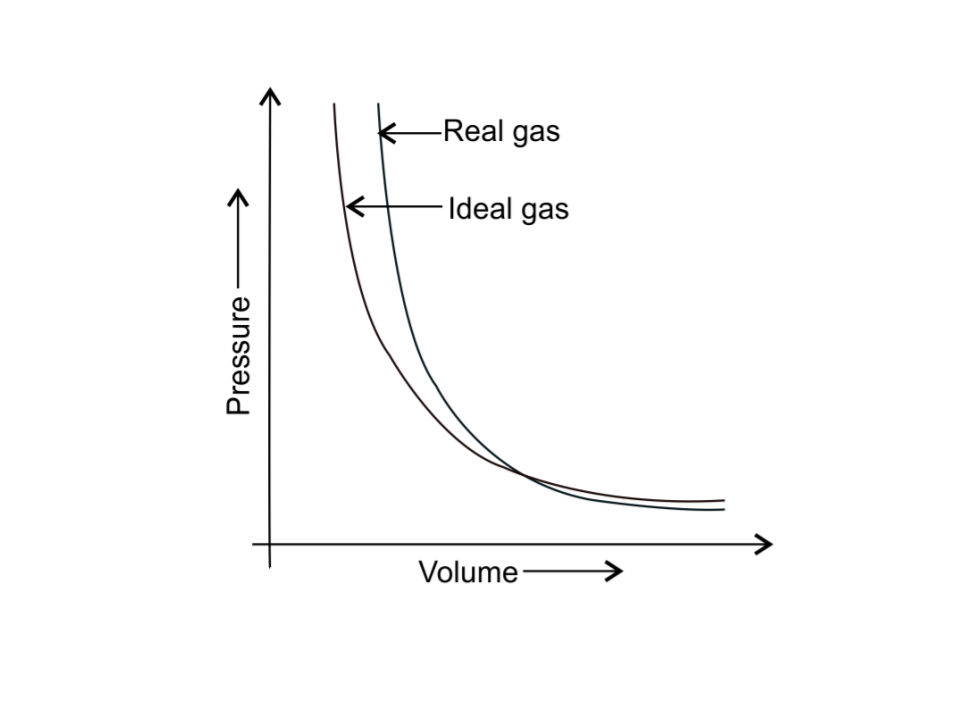Answer the following questions on the basis of this graph.

1. Interpret the behaviour of real gas with respect to ideal gas at low pressure.

Ans:  At low pressure, the curve of real gas coincides  with that of ideal gas, this shows that the deviation of  behaviour of real gas with respect to ideal gas is small or negligible.

2. Interpret the behaviour of real gas with respect to ideal gas at high pressure.

Ans: At high pressure, the curve of real gas is far apart from  ideal gas, this shows that the deviation of  behaviour of real gas with respect to ideal gas is large.

3. Mark the pressure and volume by drawing a line at the point where real gas behaves as an ideal gas.

Ans: The  pressure p1 and volume V1  are  the point where real gas behaves as an ideal gas.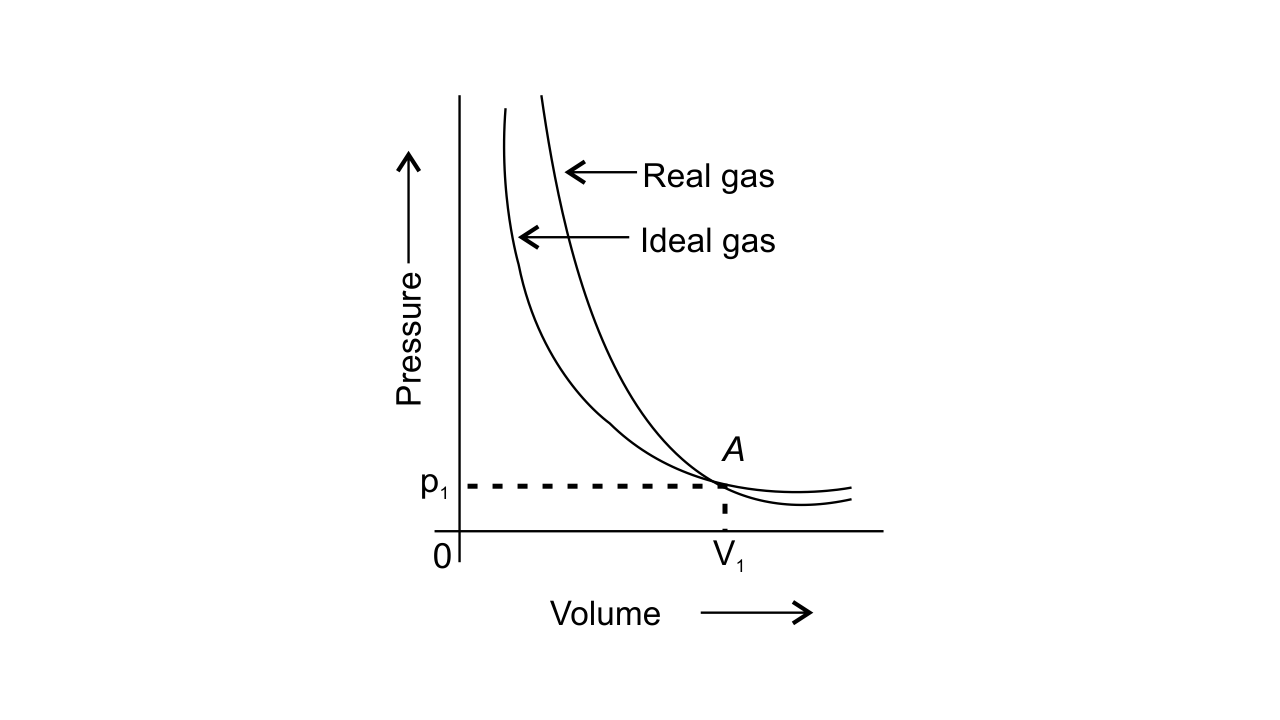### IV. Matching Type:

41. Match the graphs between the following variables with their names :

 Graphs Names (i)   Pressure vs temperature graph at constant molar volume. (a) Isotherms (ii)  Pressure vs volume graph  at constant temperature. (b) Constant temperature  curve (iii)  Volume vs temperature graph  at constant pressure. (c) Isochores (d) Isobars

Ans:

 Graphs Names (i)   Pressure vs temperature graph at constant molar volume. (c) Isochores (ii)  Pressure vs volume graph at constant temperature. (a) Isotherms (iii)  Volume vs temperature graph at constant pressure. (d) Isobars

42. Match the following gas laws with the equation representing them.

 (i)  Boyle’s law (a) V ∝ n at constant T and p (ii)  Charle’s law (b)  ptotal = p1 + p2 + p3……… at constant T, V (iii)  Dalton's law (c)   $\dfrac{PV}{T}$ = constant (iv) Avogadro law (d)  V ∝ T at constant n and p (e)   p ∝ $\dfrac{1}{V}$ at constant n and T

Ans:

 (i)  Boyle’s law (e)   p ∝ $\dfrac{1}{V}$ at constant n and T (ii)  Charle’s law (d)  V ∝ T at constant n and p (iii)  Dalton's law (b)  ptotal = p1 + p2 + p3……… at constant T, V (iv) Avogadro law (a) V ∝ n at constant T and p

43. Match the following graphs of ideal gas with their co-ordinates:

 Graphical representation x and y co-ordinates (i)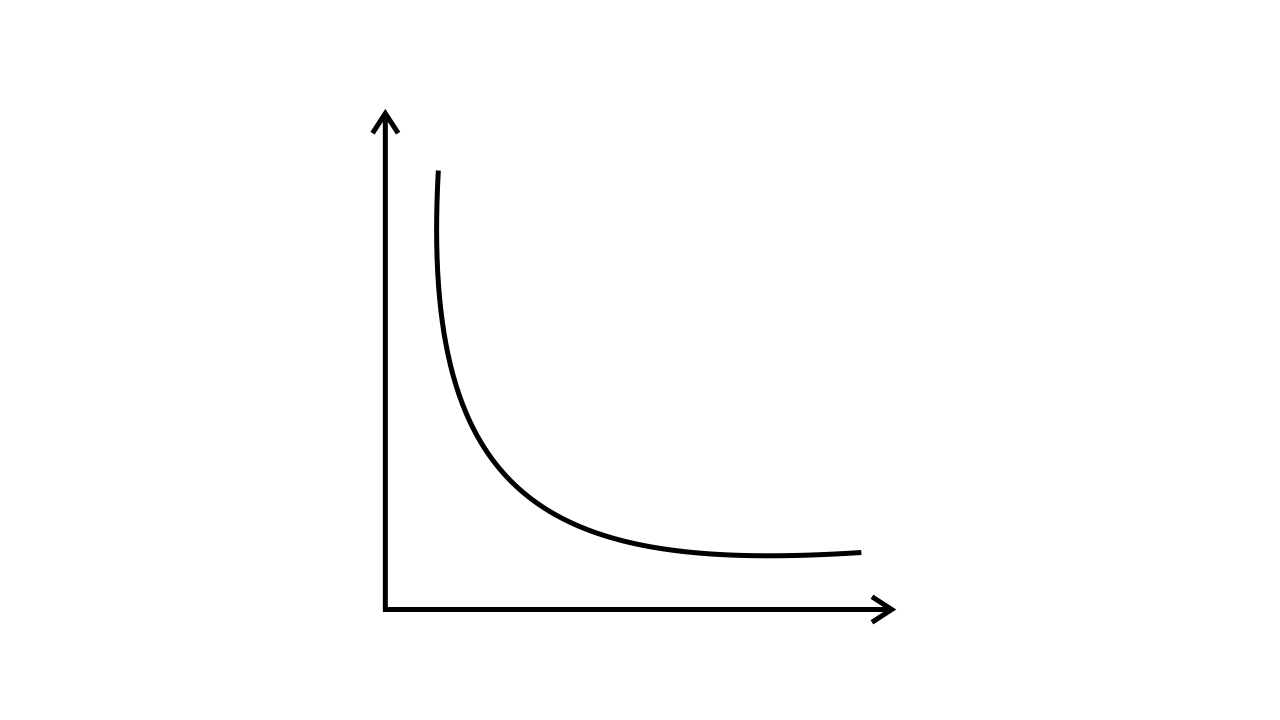(a)   pV vs. V (ii)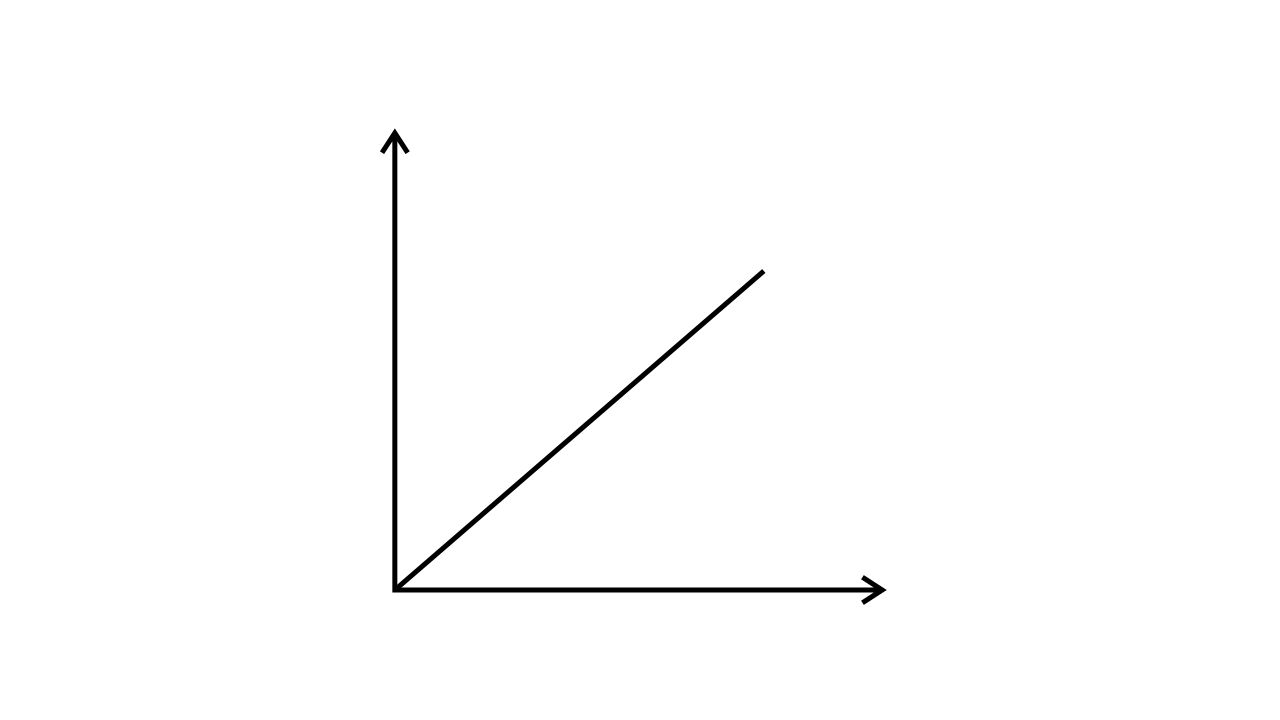(b) p vs. V (iii)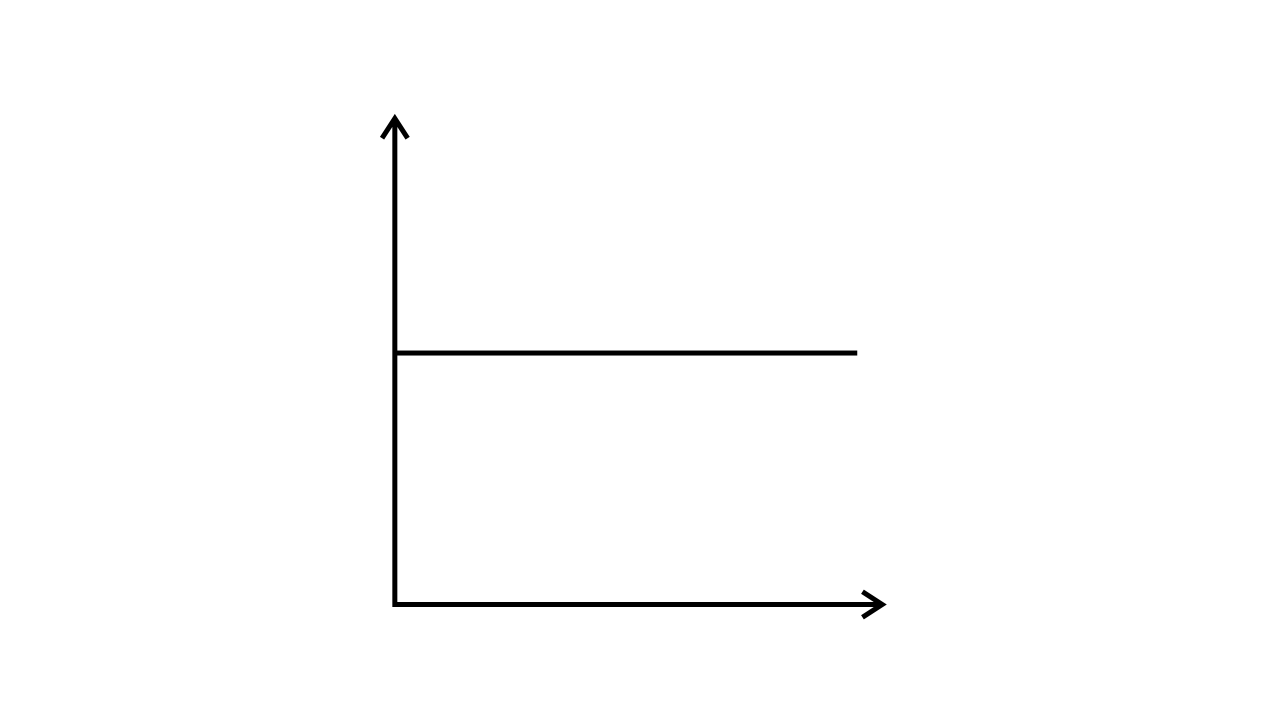(c) p vs. $\dfrac{1}{V}$

Ans:

 Graphical representation x and y co-ordinates (i)(b) p vs. V (ii)(c) p vs. $\dfrac{1}{V}$ (iii)(a)   pV vs. V

### V. Assertion and Reason Type

In the following questions a statement of Assertion (A) followed by a statement of Reason (R) is given. Choose the correct option out of the choices given below each question.

44. Assertion (A): Three states of matter are the result of balance between intermolecular forces and thermal energy of the molecules.

Reason (R): Intermolecular forces tend to keep the molecules together but    thermal energy of molecules tends to keep them apart.

i. Both A and R are true and R is the correct explanation of A.

ii. Both A and R are true but R is not the correct explanation of A.

iii. A is true but R is false.

iv. A is false but R is true.

Ans: i. Both A and R are true and R is the correct explanation of A.

45. Assertion (A): At constant temperature, pV vs V plot for real gases is not a straight line.

Reason (R) : At high pressure all gases have Z > 1 but at intermediate pressure most gases have Z < 1.

i. Both A and R are true and R is the correct explanation of A.

ii. Both A and R are true but R is not the correct explanation of A.

iii. A is true but R is false.

iv. A is false but R is true.

Ans: ii.  Both A and R are true but R is not the correct explanation of A.

At constant temperature, the pV vs V plot for real gases is not a straight line because there is intermolecular attraction present in real gases which is absent in ideal gases; hence ideal gases form a straight line in the pV vs V plot at constant temperature.

46. Assertion (A): The temperature at which vapour pressure of a liquid is equal to the external pressure is called boiling temperature.

Reason (R) : At high altitude atmospheric pressure is high.

i. Both A and R are true and R is the correct explanation of A.

ii. Both A and R are true but R is not the correct explanation of A.

iii. A is true but R is false.

iv. A is false but R is true.

Ans: iii. A is true but R is false.

At high altitude, the atmospheric pressure is low.

47. Assertion (A):  Gases do not liquefy above their critical temperature, even on applying high pressure.

Reason (R) : Above critical temperature, the molecular speed is high and intermolecular attractions cannot hold the molecules together because they escape because of high speed.

i. Both A and R are true and R is the correct explanation of A.

ii. Both A and R are true but R is not the correct explanation of A.

iii. A is true but R is false.

iv. A is false but R is true.

Ans:  i. Both A and R are true and R is the correct explanation of A.

48. Assertion (A): At critical temperature liquid passes into gaseous state imperceptibly and continuously.

Reason (R) : The density of liquid and gaseous phase is equal to critical temperature.

i. Both A and R are true and R is the correct explanation of A.

ii. Both A and Rare true but R is not the correct explanation of A.

iii. A is true but R is false.

iv. A is false but R is true.

Ans: i.  Both A and R are true and R is the correct explanation of A.

49. Assertion (A): Liquids tend to have maximum number of molecules at their surface.

Reason (R) : Small liquid drops have spherical shape.

i. Both A and R are true and R is the correct explanation of A.

ii. Both A and Rare true but R is not the correct explanation of A.

iii. A is true but R is false.

iv. A is false but R is true.

Ans: iv. A is false but R is true.

Liquid tends to minimise the number of molecules at their surface due to surface tension; this leads to the spherical shape of small liquid drops.

### VI Long Question:

50. Isotherms of carbon dioxide at various temperatures are represented in Fig. 5.5. Answer the following questions based on this figure.i. In which state will CO2 exist between the points a and b at temperature T1?

Ans:  CO2 will exist in a gaseous state between the points a and b at temperature T1

ii. At what point will CO2 start liquefying when temperature is T1?

Ans: At point b, CO2 will start liquefying when temperature is T1

iii. At what point will CO2 be completely liquefied when temperature is  T2?

Ans: At point g,  CO2 will be completely liquefied when temperature is  T2

iv. Will condensation take place when the temperature is T3.

Ans: No, the condensation will not take place  when the temperature is T3 because T3 > Tc.

v. What portion of the isotherm at T1 represents liquid and gaseous CO2 at equilibrium?

Ans:  Between  b and c of the isotherm at T1 , represents the liquid and  gaseous CO2 at equilibrium.

51. The variation of vapour pressure of different liquids with temperature is shown in Fig. 5.6.i. Calculate graphically boiling points of liquids A and B.

Ans: The  boiling points of liquid A  is approximately 315 K and of liquid B is approximately 345 K (shown below graphically).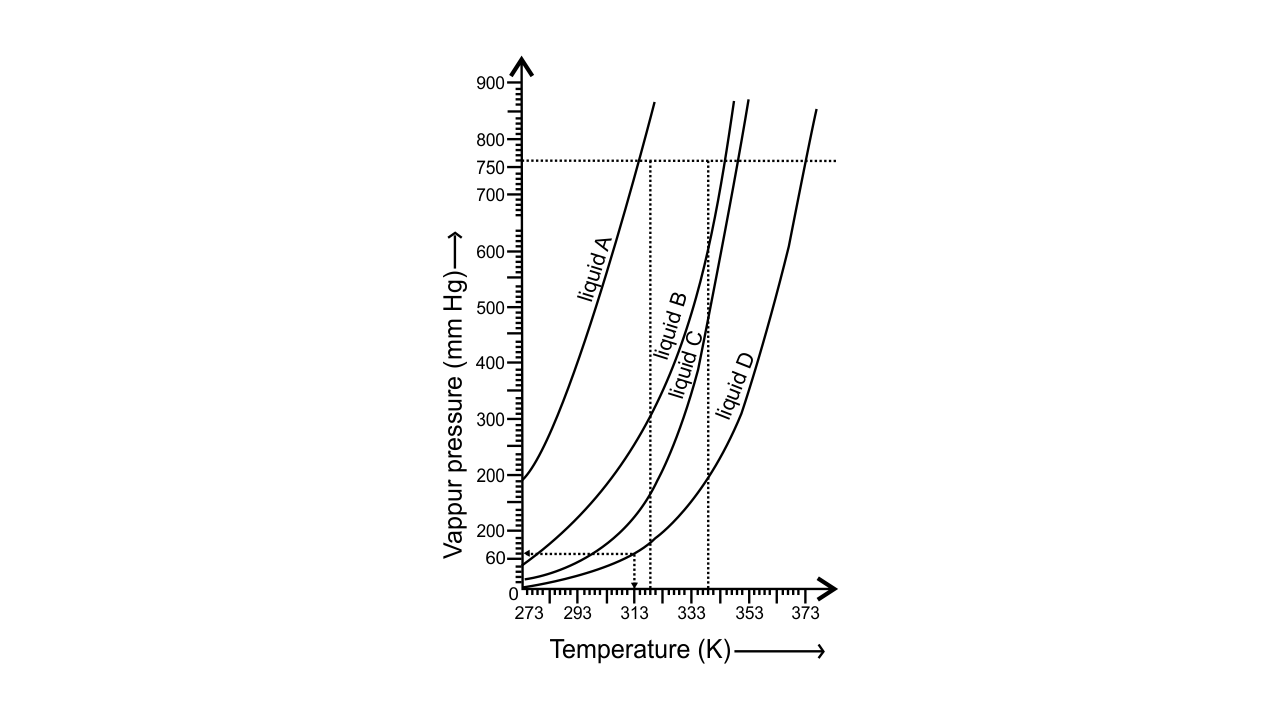ii. If we take liquid C in a closed vessel and heat it continuously. At what temperature will it boil?

Ans:  Liquid C in a closed vessel will not boil as the pressure keeps on increasing.

iii. At high altitude, atmospheric pressure is low (say 60 mm Hg). At what temperature liquid D boils?

Ans: The  liquid D will boil at approximately 313 K at high altitude when the atmospheric pressure is 60 mm Hg (shown above graphically).

iv. Pressure cooker is used for cooking food at hill stations. Explain in terms of vapour pressure why is it so?

Ans: Liquids boil when the vapour pressure becomes equal to atmospheric pressure. At hill stations, the atmospheric pressure is low at high altitude, so  water boils at low temperature.

When a pressure cooker is used, vapour pressure is increased due to which water boils at even low temperature, that too at a very short period of time.

52. Why does the boundary between liquid phase and gaseous phase disappear on heating a liquid upto critical temperature in a closed vessel? In this situation what will be the state of the substance?

Ans: When a liquid is heated in a closed vessel, the liquid changes into the vapour phase continuously. When the critical temperature is attained, the liquid changes completely into vapour phase due to which the boundary between the liquid phase and gaseous phase disappears.

53. Why does sharp glass edge become smooth on heating it upto its melting point in a flame? Explain which property of liquids is responsible for this phenomenon.

Ans: When the sharp glass edge is  heated upto its melting point in a flame, it gets melted and becomes rounded at the edge which makes it smooth. The sharp edge becomes round on melting due to the surface tension phenomenon to acquire minimum surface area.

54. Explain the term laminar flows. Is the velocity of molecules same in all the layers in laminar flow? Explain your answer.

Ans:  When liquid flows over a fixed surface, the layer of the molecule which is in the immediate contact to the surface remains stationary due to resistance or friction. The velocity of the molecules above the stationary layer increases as the distance increases from the surface. The flow in which there is gradation of velocity from one layer to the next is known as Laminar flow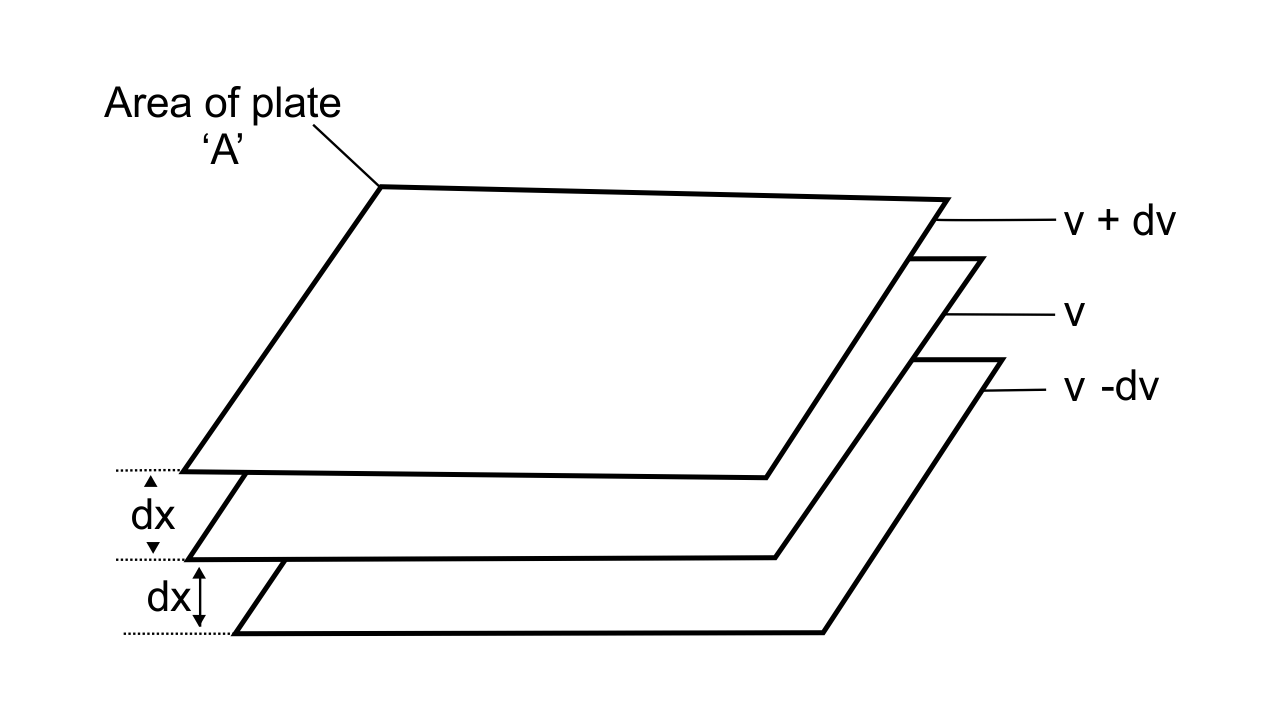Fig.: Gradation of velocity in Laminar flow

The velocity increases with increase in the distance from the surface, so the velocity of the molecules is not the same in all the layers in laminar flow.

55. Isotherms of carbon dioxide gas are shown in Fig. 5.7. Mark a path for changing gas into liquid such that only one phase (i.e., either a gas or a liquid) exists at any time during the change. Explain how the temperature, volume and pressure should be changed to carry out the change.Fig.: 5.7

Ans: The CO2 gas can be changed into liquid such that only one phase exists at any time during the change.  This can be done by vertically moving from point A to F present at the above isotherm by increasing the temperature.  Now we can  move to point G from point F  by compressing the gas along the same isotherm at the same temperature by increasing pressure.

As soon as  point G is reached, move vertically downward to point D, by decreasing the temperature. The gas CO2 will be immediately converted to liquid just below the point H (critical isotherm).  We get the liquid phase from the gaseous phase of CO2 gas without the two-phase stage.

The path is:        A $\to$ F $\to$ G $\to$  H $\to$  DFig: Path of one phase conversion of CO2 gas to liquid phase

### Benefits of Consulting NCERT Exemplar Books

• The books are designed considering the level of understanding of the Class 11 students.

• It will help the students to make last-minute preparation before appearing in the Examinations. The questions are solved with proper analysis and explanations and also elaborated with the help of short and simple techniques so that the students can grasp the idea underlying a concept.

• The books can be the best reference books that will help the students to score a good mark in their Examinations.

Since Chemistry is a research-based and knowledge-based subject, before attempting to solve the questions and answering them, the students need to emphasize the process of getting familiarised with the terminologies. Joining the free Classes on Vedantu will help them solve these issues as the expert trainers will teach them the concepts in very simple language.

The students need to be attentive and strategic while answering the Chemistry questions as a lot of equations and chemical reactions will be there to solve. Having a good grip over the knowledge of chemical compounds is extremely important for the students. Also, try to analyse and think about the equations in your mind so that you do not forget them.

Visit the website of Vedantu right now and download all the NCERT books of Chemistry to be a step ahead of others in your preparation for the Chemistry Examination.

## FAQs on NCERT Exemplar for Class 11 Chemistry Chapter-5 (Book Solutions)

1. Why is studying the States of Matter important?

The Chapter on States of Matter helps you to get familiarised with the various States of the external components that we can see daily. There are four different States of Matter that we can perceive and the Matter that we can see, generally fall under the category of four different States. They can be in solid, liquid, plasma, or gas form. You learn to identify their States and the knowledge of this practical concept is very important.

2. What does the Chapter of States of Matter deal with?

The Chapter of States of Matter that has been included in the syllabus of the students who are preparing for Class 11 Examinations is one of the most important Chapters that they are going to cover during their learning process. The Chapter deals with the various States of the external Matter, their volumes, and shapes and also with the various atoms, ions, and positions of these particles.

3. What are the types of different States of Matter?

The objects around us can generally remain present in four different forms. A Matter can be in solid form or liquid form. Matter can also be present in the form of gas and plasma. There is also the concept of a fifth State but that is not natural. The fifth State is artificially formed by human beings and it is known as the Bose-Einstein condensate. Depending on the position of the particles that a particular Matter is constituted of, it can be in any of the above-mentioned States.

4. Is understanding the concept of the States of Matter difficult?

The concept is very interesting and very easy to understand. The CBSE students get to learn about the State of Matters in Class 11 and they will find this Chapter very easy to understand if they are genuinely interested in studying Chemistry.

In this chapter, the students will get to learn about various definitions and types of atoms and various ions and electrons. They get to learn how the positions of these molecular particles decide the State of a particular Matter.

5. Is there any guidebook to consult for a better understanding of the Chemistry Chapters involving the States of Matter?

The students can take the help of the NCERT Exemplar to understand the concepts and definitions of Chemistry. There are solved question papers included in the books that will provide the students with a clear guideline of understanding the chemical equations and solving them. Referring to these books will assist the students in revising the study content as they can check out the process of solving and answering the Chemistry questions. They will also get a fair idea of the patterns of the questions and the topics they have to cover to be prepared for the Examination.